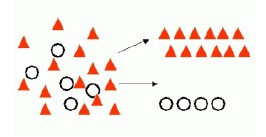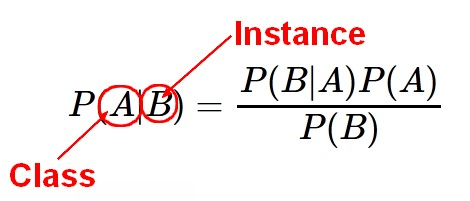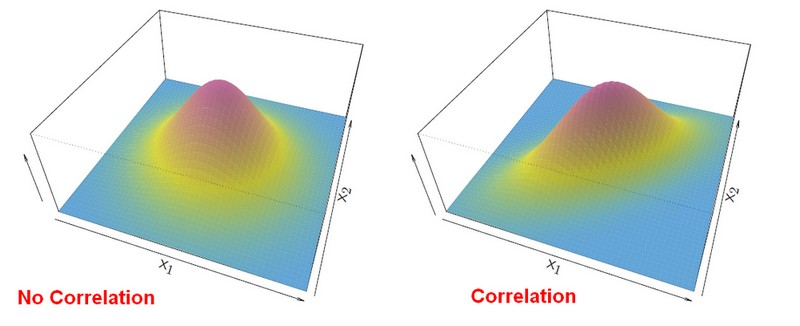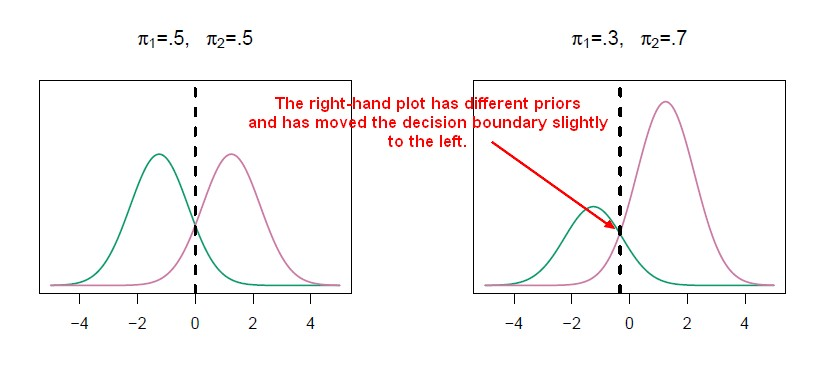# Statistical Learning - Simple Linear Discriminant Analysis (LDA)

Linear Discriminant Analysis with only one variable (p = 1).

For a generalization, see Statistics - Fisher (Multiple Linear Discriminant Analysis|multi-variant Gaussian)

## Assumption

The variance $\sigma_k$ from the distribution of the value $X_i$ when $Y_i = k$ is the same in each of the classes k.

It is an important convenience as it's going to determine whether the discriminant function that we get, the discriminant analysis, gives us linear functions or quadratic functions.

## Model Construction

### Gaussian density

The Gaussian density has the form:

$$f_k(x) = \frac{1}{\sqrt{2\pi}\sigma_k} e^-\frac{1}{2} \left (\frac{x-\mu_k}{\sigma_k} \right )^2}$$

where:

• $\mu_k$ is the mean in class k
• $\sigma_k$ is the variance in class k

### Bayes Formula

#### Total

Plugging the gaussian density into the Bayes formula, we get a rather complex expression.

$$\begin{array}{rrl} Pr(Y = k|X = x) & = & \fracPr(X = x|Y = k)  Pr(Y = k)}Pr(X = x)} \\ p_k(x) & = & \frac \pi_k \frac{1}{\sqrt{2\pi}\sigma_k} e^-\frac{1}{2} \left (\frac{x-\mu_k}{\sigma_k} \right )^2}} \sum^K_{l=1} \pi_l \frac{1}{\sqrt{2\pi}\sigma_k} e^-\frac{1}{2} \left (\frac{x-\mu_l}{\sigma_k} \right )^2}} \end{array}$$

#### Simplification

Luckily, thanks to the assumptions, there's some simplifications and cancellations.

To classify an observation to a class, we don't need to initially evaluate the probabilities. We just need to see which is the largest.

Whenever you see exponentials the first thing you want to do is take the logs.

And if you discard terms that do not depend on k, that amounts to doing a lot of cancellation of terms that don't count.

This is equivalent to assigning to the class with the largest discriminant score.

$$\delta_k(x) = x.\frac{\mu_k}{\sigma^2}-\frac{\mu_k^2}{2\sigma^2}+log(\pi_k)$$

It involves:

• x, a single variable in this case.
• the mean $\mu_k$ for the class k
• the variance $\sigma$ of the distribution
• the prior $\pi_k$ for the class k

And importantly, $\delta_k(x)$ is a linear function of x.

There's:

• a constant $\frac{\mu_k}{\sigma^2}$
• a constant term $-\frac{\mu_k^2}{2\sigma^2}+log(\pi_k)$

For each of the classes, we get one of those functions .

#### Binary

If:

• there are two classes (K = 2)
• $\pi_1 = \pi_2 = 0,5$

, you can simplify even further and see that the decision boundary is at

$$x = \frac{\mu_1 + \mu_2}{2}$$

## Parameters Estimation

• The priors are just the number in each class divided by the sample size

$$\hat{\pi_k} = \fracN_k}{N}$$

• The mean for the class k is the sum of all variable when the attribute Y is equal to the class divided by the number of case for this class

$$\hat{\mu_k} = \frac{1}{N_k}\sum_{i:y_i=k}x_i$$

The notation $\displaystyle \sum_{i:y_i=k}$ will just sum the $x_i$ 's that are in class k.

• As we're assuming that the variance is the same in each

of the classes, this formula is called a pooled variance estimate. $$\begin{array}{rrl} \hat{\sigma}^2 & = & \frac{1}{n-K}\sum_{k=1}^K\sum_{i:y_i=k}(x_i-\hat{\mu}_k)^2 \\ \end{array}$$ The formula:

• subtract from each $x_i$ the mean for its class. (the same as when we compute the variance for the class k)
• sum all those square differences.
• sum them over all the classes and then divide it by n minus k.
• estimate the sample variance separately in each of the classes and then average them in order to weight each of them. The weight has to do with how many observations were in that class relative to the total number of observations. (minus 1 and the minus k is a detail that is to do with how many parameters we've estimated for each of these estimates)

A simplified version is: $$\begin{array}{rrl} \hat{\sigma}^2 & = & \sum_{k=1}^K \frac{n_k-1}{n-K}.\hat{\sigma}^2_k \end{array}$$ where $\hat{\sigma}^2_k$ is the usual formula for the estimated variance in the kth class ie: $$\begin{array}{rrl} \hat{\sigma}^2_k & = & \frac{1}{n_k-1} \sum_{i:y_i=k} (x_i-\hat{\mu_k})^2 \end{array}$$

Recommended PagesData Mining - (Classifier|Classification Function)

A classifier is a Supervised function (machine learning tool) where the learned (target) attribute is categorical (“nominal”) in order to classify. It is used after the learning process to classify...Data Mining - (Discriminative|conditional) models

Discriminative models, also called conditional models, are a class of models used in machine learning for modeling the dependence of an unobserved variable y on an observed variable x. Discriminative...Data Mining - Naive Bayes (NB)

Naive Bayes (NB) is a simple supervised function and is special form of discriminant analysis. It's a generative model and therefore returns probabilities. It's the opposite classification strategy...R - Linear Discriminant Analysis (LDA)

Linear Discriminant Analysis in R Fit the model Print it by tapping its name where: the prior probabilities are just the proportions of false and true in the data set. It's kind of a random...Statistics - Fisher (Multiple Linear Discriminant Analysis|multi-variant Gaussian)

multi-variant Gaussians. Fisher has describe first this analysis with his Iris Data Set. A Fisher's linear discriminant analysis or Gaussian LDA measures which centroid from each class is the closest....Statistics Learning - Discriminant analysis

Discriminant analysis is a classification method. In discriminant analysis, the idea is to: model the distribution of X in each of the classes separately. use what's known as Bayes theorem to flip...